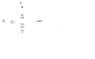# Graph Vo vs Vin: Zener Diode Behavior

• nwilkins259

#### nwilkins259

There is a variable Vin which goes through a resistor to a node (Vo) which then branches through a diode to a 3V source or to ground through a Zener diode.

I'm to sketch the graph of Vo as a function of Vin.

-4V < Vin < 4V
The resistor is 1K
The diode is CVD model, Von is .7V,
Vzener = 2.7V and Rzener = 100Ω

a picture is attached.

Can current flow through the Zener diode in both directions?

for example when Vin = -4V through -.7V will the zener diode operate like a normal diode?

and then cease operation until the reverse breakdown voltage of 2.7 through 3.7 volts is reached?

at 3.7 volts i assume all current would flow through the normal diode on top due to Rzener.

#### Attachments

•ZenerProblem.png
1.2 KB · Views: 538
There is a variable Vin which goes through a resistor to a node (Vo) which then branches through a diode to a 3V source or to ground through a Zener diode.

I'm to sketch the graph of Vo as a function of Vin.

-4V < Vin < 4V
The resistor is 1K
The diode is CVD model, Von is .7V,
Vzener = 2.7V and Rzener = 100Ω

a picture is attached.

Can current flow through the Zener diode in both directions?

for example when Vin = -4V through -.7V will the zener diode operate like a normal diode?

and then cease operation until the reverse breakdown voltage of 2.7 through 3.7 volts is reached?

at 3.7 volts i assume all current would flow through the normal diode on top due to Rzener.

Yes, current can flow in bot directions since the zener in the back direction looks like a reular diode, forward-biased.

EDIT: oops, not quite everything right.

A t some + input voltage Vin, the regular diode anode voltage Vd will exceed +3.7V and conduct. Any further increase in Vin will result in all of the ADDITIONAL current diverted thru the regular diode.

The point at which the regular diode starts to conduct is found from

(Vin - Vd)G1 = (Vd - Vz)G2 when Vd = +3.7V.

G1 = 1/1KΩ
G2 = 1/100Ω
Vz = 2.7V

Last edited:
Can current flow through the Zener diode in both directions?
Yes.

for example when Vin = -4V through -.7V will the zener diode operate like a normal diode?
Precisely.

at 3.7 volts i assume all current would flow through the normal diode on top due to Rzener.
Not so. (I doubt that you mean what you wrote here.)